## Introduction

Quasiparticle (QP) excitations adversely affect the performance of superconducting devices in a wide range of applications. They limit the sensitivity of photon detectors in astronomy1,2, the accuracy of current sources in metrology3, the cooling power of micro-refrigerators4 and could break the topological protection of Majorana qubits5. In superconducting quantum information processing (QIP), the preservation of charge parity (even or odd number of electrons) has historically been a primary concern. In the first superconducting qubit, termed the Cooper-pair box (CPB)6, maintaining the parity in a small island connected to a reservoir via Josephson junctions is essential to qubit operation. The qubit states |0〉 and |1〉 consist of symmetric superpositions of charge states of equal parity, brought into resonance by a controlled charge bias ng and split by the Josephson tunnelling energy EJ (EC, the island Cooper-pair charging energy). QP tunnelling across the junction changes the island parity, ‘poisoning’ the box until parity switches back or ng is offset by ±e (ref. 7). QP poisoning has been extensively studied in CPBs and similar devices, such as single-Cooper-pair transistors and charge pumps, with most experiments8,9,10,11,12,13 finding parity switching times of 10 μs–1 ms, and some >1 s (refs 14, 15, 16). While these times are long compared with qubit gate operations (10 ns), the sensitivity of the CPB qubit transition frequency ω01 to background charge fluctuations limits the dephasing time to <1 μs, severely restricting the use of traditional CPBs in QIP.

Engineering the CPB into the transmon regime EJEC (refs 17, 18) exponentially suppresses the sensitivity of ω01 to charge-parity and background charge fluctuations. However, recent theory19,20,21 predicts that QP tunnelling remains a relevant source of relaxation and pure dephasing of the qubit degree of freedom. The contribution of QP tunnelling on qubit decoherence has become particularly interesting as control of the Purcell effect22 in circuit quantum electrodynamics (cQED)23 and the reduced contribution of dielectric losses in three-dimensional geometries24 have allowed reaching the 100 μs scale. To guide further improvements, it is imperative to precisely pinpoint the timescale for QP tunnelling and its contribution to qubit decoherence. To date, only upper and lower bounds on QP tunnelling rates have been placed18,25 in transmon qubits, while the effect of QP tunnelling on transmon decoherence remains unexplored.

Here, we transform a state-of-the-art single-junction transmon qubit into a real-time charge-parity detector. We measure both the characteristic time for QP tunnelling across the junction and the effect of such tunnelling on qubit decoherence at the millisecond timescale. Our qubit is controlled and measured in a three-dimensional cQED architecture24, an emerging platform for QIP, without need for any electrometer or other circuitry. At the heart of our detection scheme is a very small but detectable parity dependence of the qubit transition frequency (up to 0.04% of the average ω01/2π=4.387 GHz), obtained by choosing EJ/EC=25.

## Results

### Evidence of QP tunnelling

Standard Ramsey fringe experiments provide the first evidence of QP tunnelling across the qubit junction, as shown in Fig. 1 for a refrigerator temperature Tr=20 mK. Instead of the usual single decaying sinusoid, we observe two. Repeated Ramsey experiments always reveal two frequencies, fluctuating symmetrically about the average ω01 (Fig. 1c). The double frequency pattern results from QP tunnelling events causing ng to shift by ±e. The fluctuation in the difference Δf between the two frequencies is owing to background charge motion slow compared with QP tunnelling. The observation of two frequencies in every experiment shows that QP tunnelling is fast compared with the averaging time (15 s), but slow compared with the maximum time 1/2Δf5 μs (ref. 26). From the similar amplitude of the sinusoids, we can already deduce that the two parities are equally likely. Clearly, these time-averaged measurements only loosely bound the timescale for QP tunnelling, similarly to refs 18, 25.

### Real-time detection of charge-parity fluctuations

In order to accurately pinpoint the timescale for QP tunnelling, we have devised a scheme to monitor the charge parity in real time using the qubit itself (Fig. 2a). The scheme takes advantage of recent developments in high-fidelity nondemolition readout27,28 and feedback control29. Starting from |0〉, the qubit is prepared in the superposition state (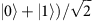with a π/2 y-pulse at ω01. The Rabi frequency of 16 MHz is sufficient to drive both odd- and even-parity qubit transitions, which differ by 2Δf ≤1.76 MHz. The qubit then acquires a phase ±π/2 during a chosen idle time Δt=1/4Δf, where the + (−) sign corresponds to even (odd) parity. A second π/2 x-pulse completes the mapping of parity into a qubit basis state, even →|0〉, odd →|1〉. A following projective qubit measurement ideally matches the result P=1 (−1) to even (odd) parity. Feedback-based reset29 reinitializes the qubit to |0〉 and allows repeating this sequence every Δtexp=6 μs.Figure 2: Real-time measurement of QP tunnelling using the transmon qubit as its own charge-parity detector.

The time evolution of charge parity is encoded in the series of results P (Fig. 2b). The time series has zero average, confirming that the two charge parities are equally probable. Both the QP dynamics and the detection infidelity determine the distribution of dwell times t+1 and t−1 (Fig. 2d). The measured identical histograms match a numerical simulation of a symmetric random telegraph signal (RTS) with transition rate Γrts, masked by uncorrelated detection errors occurring with probability (1−F)/2. These two noise processes contribute distinct signatures to the spectral density of P (Fig. 2c). The best fit of the form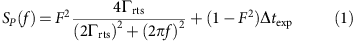shows excellent agreement, giving 1/Γrts=0.79 ms and F=0.92.

### Measurement of QP-tunnelling-induced qubit decoherence

While the above scheme detects a characteristic time for QP tunnelling, our goal is to determine the effect of such QP tunnelling on the performance of the qubit degree of freedom. Specifically, we aim to determine the rates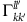connecting level |kl〉 to level |kl′〉 (k (k′) and l (l′) denote the initial (final) qubit and parity state, respectively, as illustrated in Fig. 3b). For example,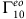denotes the QP-tunnelling-induced qubit relaxation rate. Based on the identical distribution of dwell times, we safely approximate symmetric rates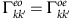.Figure 3: Effect of QP tunnelling on the qubit degree of freedom.

To extract the above rates, we measure the autocorrelation function of charge parity, conditioned on specific initial and final qubit states (Fig. 3). We first execute the charge-parity sequence illustrated in Fig. 2. Conditioning on the result of the projective measurement P1=+1 postselects the qubit in |0〉 and even parity. After a waiting time τ, another measurement M determines the qubit state. Conditioning also on M=+1 ensures that the qubit ends in |0〉. A second instance of the charge-parity sequence, ending with P2, completes the scheme. The average result, once corrected for detector infidelity (see Methods), is the parity autocorrelation R00(τ)=〈P(0)P(τ)〉00, with first (second) subscript indicating initial (final) qubit state. Neglecting qubit excitation, that is, setting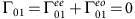, R00(τ) simply decays as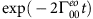. The exact solution shows that this remains a valid approximation when including the measured Γ01=1/6 ms−1, as the probability of multiple qubit transitions in τ is negligible. Similarly, we measure the parity autocorrelation with qubit initially and finally in |1〉,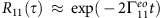. To do this, we use the same conditioning, but apply a π pulse after P1 and before M. Exponential decay fits give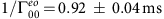and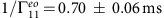.

To quantify the contribution of QP tunnelling to the measured net qubit relaxation time T1=1/Γ10=0.14±0.01 ms (see Methods), we apply the same method, but condition on initial state |1〉 and final state |0〉. The ratio of QP-induced to total relaxation rates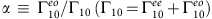can be extracted from R10(τ→0)=1−2α. The best fit of the model R10(τ) to the data, with α as only free parameter, gives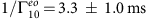and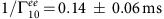. This result clearly demonstrates that QP tunnelling does not dominate qubit relaxation at Tr=20 mK, contributing only 5% of qubit relaxation events.

To facilitate comparison of the measured rates to theory, we perform the above experiments at elevated Tr (Fig. 4). We observe that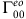,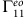andhave similar magnitude and jointly increase with Tr in the range 20–170 mK. However, T1 remains insensitive to Tr until 150 mK. The observed sign reversal in R10(τ→0) near this temperature (Fig. 4b) indicates that QP tunnelling becomes the dominant qubit relaxation process.Figure 4: Temperature dependence of QP tunnelling contribution to qubit relaxation and dephasing.

## Discussion

The effect of QP dynamics on the qubit degree of freedom in superconducting circuits has been extensively studied theoretically7,19,20,21. For transmon qubits, the predicted QP-induced relaxation rate is19,20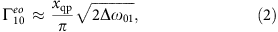where xqp=nqp/2ν0Δ is the QP density nqp normalized to the Cooper-pair density, with ν0=1.2 × 104 μm−3μeV−1 the single-spin density of states at the Fermi energy11 and Δ the Al superconducting gap. This relation holds for any energy distribution of QPs. For Tr≥150 mK, the data closely match equation (2) using the thermal equilibrium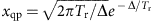and Δ=170 μeV, the value estimated from the normal-state resistance of the junction (see Methods). The suppression ofat lower Tr is much weaker than expected from a thermal QP distribution. Using equation (2), we estimate nqp=0.04±0.01 μm−3 at Tr=20 mK, matching the lowest value reported for Al in a Cooper-pair transistor for use in metrology30. Improved shielding against infrared radiation31 could further decrease nqp at low Tr, consequently suppressing the contribution of QP tunnelling to qubit relaxation, and will be pursued in future work.

QP tunnelling events that do not induce qubit transitions still contribute to pure qubit dephasing. Calculations based on refs 19, 21 predict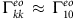, in good agreement with the data (Fig. 4c). It is presently not understood whether such QP tunnelling events completely destroy qubit superposition states (case A) or simply change the qubit precession frequency (case B). In either case, in the regime of strongly coupled RTS valid for our experiment (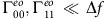(ref. 26)) the QP-induced dephasing time is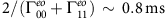. For case B, this time would further increase in the weak-coupling regime (attained at EJ/EC60) owing to motional averaging26.

In conclusion, we have converted a state-of-the-art transmon qubit into its own charge-parity detector to answer whether QP tunnelling already limits qubit coherence. We measure the contribution of QP tunnelling to relaxation and dephasing to be in the millisecond range. We stress that these times are directly measured, without relying on any theory. Thus, transmon qubit coherence can increase by at least another order of magnitude before QP tunnelling begins to limit coherence. Such an increase would facilitate the realization of fault-tolerant quantum computing in the solid state. The implemented scheme also provides an essential ingredient in the envisioned top-transmon architecture for manipulation and readout of Majorana qubits32.

## Methods

### Device parameters

The transmon has Josephson energy EJ=8.442 GHz and charging energy EC=0.334 GHz. Using the Ambegaokar–Baratoff relation EJRn=Δ/8e2 and the measured room-temperature resistance Rn,300K=15.2 kΩ of the single Josephson junction, we estimate Δ=170 μeV. The qubit couples to the cavity fundamental mode ωr/2π=6.551 GHz (decay rate κ/2π=720 kHz) with strength g/2π=66 MHz, inducing a dispersive shift χ/π=−1.0 MHz. The qubit relaxation time T1 may be limited by the multi-mode Purcell effect22. A simple estimate including only the fundamental mode gives 240 μs. The dephasing time,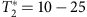μs, is limited by background charge fluctuations (see Supplementary Fig. S1).

### Experimental setup

The device and the experimental setup are similar to those described in refs 28, 29. Here, we detail the changes we made since these earlier reports. In an effort to lower the transmon residual excitation, we replaced the Al cavity with a Cu cavity33, improved thermal anchoring to the mixing chamber plate and added low-pass filters (K&L Microwave 6L250-8000/T18000-O/O) on the input and output ports of the cavity. As a result, the transmon effective temperature decreased from 127 to 55 mK, corresponding to a reduction of total steady-state excitation from 16 to 2%, respectively. As these changes were made simultaneously, we cannot pinpoint the individual contributions to the improved thermalization.Projective readout with 99% fidelity is achieved by homodyne detection with a 400 ns pulse at ωrχ, aided by a Josephson parametric amplifier28. To perform qubit reset faster, we replaced the ADwin processor with a home-built feedback controller based on a complex programmable logic device (CPLD, Altera MAX V). The CPLD integrates the last 200 ns of the readout signal and conditionally triggers a π pulse (all resonant pulses are Gaussian, with σ=8 ns, and total duration 32 ns). The CPLD allows a response time, from the end of signal integration to the π-pulse trigger, of 0.11 μs. The total loop time, from the start of the measurement pulse to the end of the triggered π pulse at the cavity input, is 0.98 μs. However, a delay is added to reach 2 μs (10/κ) between the end of measurement and the start of the conditioned π pulse, ensuring that the cavity is devoid of readout photons.

### Extraction of QP tunnelling rates

To convert 〈P2(τ)〉kk into Rkk(τ), we correct for the overall detection errors, distributed among readout (<1%) and reset (1%) infidelities, suboptimal Δt (<2%) and dephasing during Δt (remaining 1−3%). For this correction, we first fit an exponential decay to 〈P2(τ)〉00 and 〈P2(τ)〉11. The average of the best-fit value at τ=0 is used to renormalize the data in Figs 3c and 4a. The fitted decay times are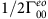and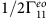, respectively. To extractand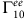, we fit the solution of equation (2) to R10(τ), using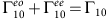. Γ10 is obtained from the equilibration time Teq after inverting the steady-state populations P|0〉,ss, P|1〉,ss with a π pulse: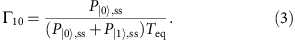The total excitation 1−P|0〉,ss is obtained by measurement and postselection29. Equation (3) remains a valid approximation even for the highest temperatures in Fig. 4, at which population of higher excited states becomes relevant. In this case, the populations P|0〉,ss, P|1〉,ss are estimated from the total excitation, assuming that the populations are thermally distributed29. Error bars for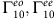are calculated from the s.d. of repeated T1 measurements and the fit uncertainty in α.

### Validation of the charge-parity detector

We perform several control experiments to validate the use of the qubit as a charge-parity detector. First, the parity to qubit-state conversion is tested with suboptimal choices of the Ramsey interval Δt (Supplementary Fig. S2). As expected from equation (1), the white noise level in SP increases at the expense of the signal contrast as Δt deviates from the optimal choice 1/4Δf. Remarkably, the extracted rate Γrts is approximately constant down to F0.4. This is consistent with the model of charge parity as a symmetric RTS, with time constant determined solely by QP tunnelling.In a second test, we replace the Ramsey-like sequence with a single pulse, with rotation angle θ. Time series of M for θ=0, π and π/2 are shown in Supplementary Fig. S3a. The very high occurrence (99%) of 1 (−1) for θ=0 (π) equals the efficiency of reset, following each measurement M. For θ=π/2, the qubit is repeatedly prepared in an equal superposition of |0〉 and |1〉, and the measurement produces uncorrelated projection noise. The spectra of these control experiments are compared with the QP tunnelling measurement in Supplementary Fig. S3b, clearly showing that the observed RTS is owing to the signal acquired during Δt.As a final test of the charge-parity detector, we subject the qubit to an externally generated RTS, similar to ref. 25. Symmetric RTS sequences with switching rate Γπ are generated in LabVIEW and sent to an ADwin controller. The ADwin samples the RTS at 9 μs interval. When the signal is +1, the ADwin triggers an AWG520 (also used for reset29), which then applies a π pulse on the qubit. As a result, the measured qubit state in M is conditioned on the RTS state, mimicking the parity-controlled π pulse implemented in Fig. 2. In all cases, the fitted rates Γfit match the programmed Γπ within 3% (Supplementary Fig. S4).